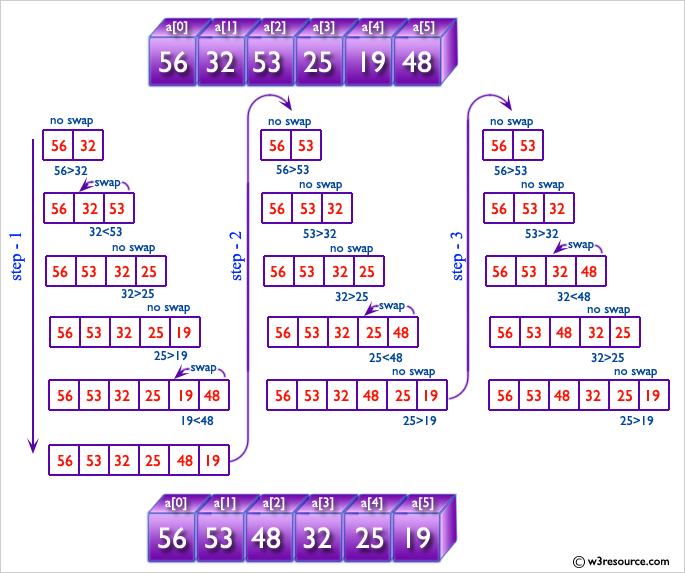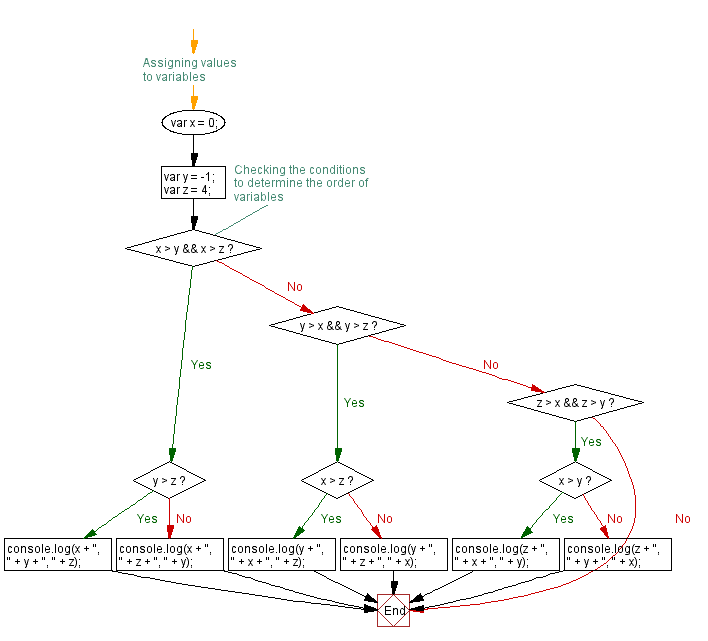# JavaScript: How to sort three numbers

## JavaScript Conditional Statement and loops: Exercise-3 with Solution

Write a JavaScript conditional statement to sort three numbers. Display an alert box to show the result.

Sample numbers: 3, -7, 2
Output : The sign is -

Pictorial Presentation:Sample Solution:-

HTML Code:

``````<!DOCTYPE html>
<html>
<meta charset=utf-8 />
<title>JavaScript conditional statement to sort three numbers</title>
<body>

</body>
</html>
```
```

JavaScript Code:

``````var x= 0;
var y=-1;
var z= 4;
if (x>y && x>z)
{
if (y>z)
{
console.log(x + ", " + y + ", " +z);
}
else
{
console.log(x + ", " + z + ", " +y);
}
}
else if (y>x && y >z)
{
if (x>z)
{
console.log(y + ", " + x + ", " +z);
}
else
{
console.log(y + ", " + z + ", " +x);
}
}
else if (z>x && z>y)
{
if (x>y)
{
console.log(z + ", " + x + ", " +y);
}
else
{
console.log(z + ", " + y + ", " +x);
}
}
```
```

Sample Output:

```4, 0, -1
```

Flowchart:Live Demo:

See the Pen javascript-conditional-statements-and-loops-exercise-3 by w3resource (@w3resource) on CodePen.

Improve this sample solution and post your code through Disqus

What is the difficulty level of this exercise?

﻿

New Content: Composer: Dependency manager for PHP, R Programming